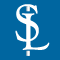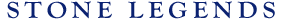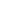HOMEPRODUCTSIDEA CENTERSERVICESTECHNICALABOUT USHello Guest Login  |  Become Member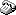800-398-1199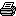214-398-1293MapEmail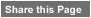Click to Share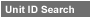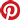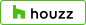Select which variables you would like to search by.Type DescriptionPrimary ViewPrimary ModifierUnit NameView Definitions

View Definitions

View DefinitionsX-Dim Y-Dim Z-Dim XX-Dim YY-Dim ZZ-Dim R-Dim D-Dim P-Dim
 Click column heading to re-sort results list.Product Name Primary View Modifier Pri Dimx  y  z Alt Dimxx  yy  zz Alt2 Dimr  d  p E\$timated Thumbnail Aether1 Bracket Rectangular Corbel X = 15-5/8" Y = 9-1/4"Z = 11-1/2" XX = n/a YY = n/aZZ = 4-1/2" R = n/a D = n/aP = n/a \$ 68.18Aether2 Bracket Rectangular Corbel X = 15-3/4" Y = 5-1/8"Z = 8" XX = n/a YY = n/aZZ = 0-7/8" R = n/a D = n/aP = n/a \$ 38.48Aether3 Bracket Rectangular Corbel X = 15-1/2" Y = 9-1/4"Z = 8" XX = n/a YY = n/aZZ = 1" R = n/a D = n/aP = n/a \$ 66.96Aether4 Bracket Rectangular Corbel X = 18" Y = 7-5/8"Z = 8-7/8" XX = n/a YY = n/aZZ = 4-1/4" R = n/a D = n/aP = n/a \$ 68.85Aether5 Bracket Rectangular Corbel X = 16-1/2" Y = 9"Z = 11-1/4" XX = n/a YY = n/aZZ = 3-5/8" R = n/a D = n/aP = n/a \$ 99.23Bracket-Split Bracket X = 50-3/8" Y = 4-13/16"Z = 26-3/8" XX = n/a YY = n/aZZ = 24-1/4" R = n/a D = n/aP = n/a \$ 999999.00Branelli Bracket SpecialShape X = 39-1/2" Y = 13-1/2"Z = 16-1/4" XX = n/a YY = n/aZZ = n/a R = n/a D = n/aP = n/a \$ 739.20Breton1 Bracket Rectangular Ornament X = 24-1/2" Y = 9"Z = 20" XX = n/a YY = n/aZZ = 8-1/2" R = n/a D = n/aP = n/a \$ 153.90Corinth1 Bracket Rectangular Ornament X = 26-3/4" Y = 22-1/4"Z = 7-1/2" XX = n/a YY = n/aZZ = 2" R = n/a D = n/aP = n/a \$ 136.28Enola Bracket SpecialShape X = 28-1/4" Y = 11-1/4"Z = 12-3/8" XX = n/a YY = n/aZZ = n/a R = n/a D = n/aP = n/a \$ 327.60Festa Imperial Bracket Rectangular Corbel X = 15-1/8" Y = 15-1/2"Z = 14-3/4" XX = n/a YY = 10"ZZ = 12" R = n/a D = n/aP = n/a \$ 164.53Festa Roma Bracket Rectangular Corbel X = 15-3/8" Y = 10"Z = 4-3/4" XX = n/a YY = n/aZZ = 2" R = n/a D = n/aP = n/a \$ 29.08Fiore Bracket Bracket X = 24" Y = 59-3/4"Z = 12" XX = n/a YY = n/aZZ = n/a R = n/a D = n/aP = n/a \$ 999999.00Flauta1 Bracket Rectangular X = 32-1/4" Y = 10"Z = 20" XX = n/a YY = n/aZZ = 5" R = n/a D = n/aP = n/a \$ 237.60Fretted Leaf Scroll Bracket Rectangular Ornament X = 13-1/4" Y = 6-1/4"Z = 5-1/2" XX = n/a YY = n/aZZ = n/a R = n/a D = n/aP = n/a \$ 84.62Grande Scroll 1 Bracket X = 19" Y = 19"Z = 5-1/2" XX = n/a YY = n/aZZ = n/a R = n/a D = n/aP = n/a \$ 999999.00Grande Volute1 Bracket SpecialShape Volute X = 22-3/4" Y = 18-1/8"Z = 12" XX = n/a YY = n/aZZ = n/a R = n/a D = n/aP = n/a \$ 288.55Grande Volute2 Bracket Rectangular Volute X = 18-7/8" Y = 16-1/4"Z = 15-7/8" XX = n/a YY = n/aZZ = n/a R = n/a D = n/aP = n/a \$ 201.66Malena Bracket Rectangular X = 17-13/16" Y = 11-11/16"Z = 9-3/4" XX = n/a YY = 3-7/8"ZZ = 5-13/16" R = n/a D = n/aP = n/a \$ 999999.00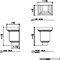Milani 2 Bracket X = 11-3/4" Y = 21-1/8"Z = 12" XX = n/a YY = n/aZZ = n/a R = n/a D = n/aP = n/a \$ 999999.00Milani1 Bracket Rectangular Volute X = 23-1/4" Y = 10-1/2"Z = 35-3/4" XX = n/a YY = 8"ZZ = n/a R = n/a D = n/aP = n/a \$ 362.86Mitra Bracket SpecialShape Volute X = 18-1/2" Y = 7-1/2"Z = 7-11/16" XX = n/a YY = n/aZZ = 4" R = n/a D = n/aP = n/a \$ 999999.00Montague1 Bracket X = 12" Y = 6"Z = 24" XX = n/a YY = n/aZZ = n/a R = n/a D = n/aP = n/a \$ 999999.00Olinda1 Bracket Rectangular X = 10" Y = 10"Z = 15-1/2" XX = n/a YY = n/aZZ = 6" R = n/a D = n/aP = n/a \$ 71.10Ontogeny Bracket 1 Bracket X = 12" Y = 10"Z = 24" XX = n/a YY = n/aZZ = 16" R = n/a D = n/aP = n/a \$ 999999.00Ontogeny Bracket 2 Bracket X = 10" Y = 6"Z = 12" XX = n/a YY = n/aZZ = 4" R = n/a D = n/aP = n/a \$ 999999.00Precade1 Bracket X = 15-5/8" Y = 6"Z = 15" XX = n/a YY = 4"ZZ = 4" R = n/a D = n/aP = n/a \$ 54.05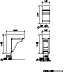Prelude1 Bracket SpecialShape Volute X = 28-7/8" Y = 28-7/8"Z = 3-1/2" XX = n/a YY = n/aZZ = 2-1/2" R = n/a D = n/aP = 2-1/2" \$ 205.20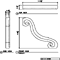Prelude2 Bracket SpecialShape Volute X = 18-7/8" Y = 18"Z = 3-1/2" XX = n/a YY = n/aZZ = 2-1/2" R = n/a D = n/aP = 2-1/2" \$ 88.73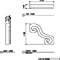Roselli1 Bracket Rectangular Corbel X = 9-1/2" Y = 7-3/4"Z = 6-1/4" XX = n/a YY = 4-3/4"ZZ = n/a R = n/a D = n/aP = n/a \$ 25.60Roselli2 Bracket Rectangular Corbel X = 9-11/16" Y = 7-3/4"Z = 7-5/8" XX = n/a YY = n/aZZ = n/a R = n/a D = n/aP = 4-3/4" \$ 34.40Scylla1 Bracket SpecialShape X = 10-3/8" Y = 16-1/8"Z = 10" XX = n/a YY = n/aZZ = 5-1/2" R = n/a D = n/aP = n/a \$ 60.68Sorvino1 Bracket Rectangular X = 24-9/16" Y = 5"Z = 9-5/8" XX = n/a YY = n/aZZ = 5-3/16" R = n/a D = n/aP = n/a \$ 58.06Stroble Corbel Bracket Rectangular X = 15-1/2" Y = 9-1/4"Z = 10-1/2" XX = n/a YY = n/aZZ = n/a R = n/a D = n/aP = n/a \$ 68.18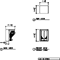Triton1 Bracket Bracket Rectangular Volute X = 16" Y = 28-5/8"Z = 15" XX = n/a YY = n/aZZ = 2" R = n/a D = n/aP = n/a \$ 999999.00Vala Bracket SpecialShape Volute X = 3" Y = 12"Z = 5-7/8" XX = n/a YY = n/aZZ = n/a R = n/a D = n/aP = n/a \$ 999999.00Valverde Bracket SpecialShape X = 39-7/8" Y = 19-7/16"Z = 6" XX = n/a YY = n/aZZ = 5" R = n/a D = n/aP = n/a \$ 355.68Vanya Bracket SpecialShape Volute X = 6-1/4" Y = 4-1/2"Z = 7-5/16" XX = n/a YY = n/aZZ = 4" R = n/a D = n/aP = n/a \$ 999999.00Vedette Bracket SpecialShape Volute X = 12" Y = 9"Z = 16" XX = n/a YY = n/aZZ = n/a R = n/a D = n/aP = n/a \$ 999999.00Vedette1 Bracket SpecialShape Volute X = 21-1/2" Y = 7-1/2"Z = 16" XX = n/a YY = n/aZZ = n/a R = n/a D = n/aP = n/a \$ 999999.00Visby 2 Bracket Rectangular X = 19-13/16" Y = 10-7/8"Z = 6" XX = n/a YY = n/aZZ = 5-5/8" R = n/a D = n/aP = n/a \$ 999999.00Visby1 Bracket Rectangular Volute X = 24" Y = 11-7/8"Z = 12" XX = n/a YY = 10-1/8"ZZ = n/a R = n/a D = n/aP = n/a \$ 113.76Vladimir Bracket Rectangular Volute X = 18-7/8" Y = 16-1/4"Z = 15-7/8" XX = n/a YY = n/aZZ = n/a R = n/a D = n/aP = n/a \$ 194.88Volare1 Bracket Rectangular Corbel X = 21-1/2" Y = 9-3/4"Z = 21-1/2" XX = n/a YY = n/aZZ = n/a R = n/a D = n/aP = n/a \$ 126.12Volte1 Bracket Rectangular Corbel X = 24-1/2" Y = 10-1/8"Z = 16-3/4" XX = n/a YY = 9"ZZ = 5-1/2" R = 6" D = n/aP = n/a \$ 202.50WildRose1 Bracket Rectangular Ornament X = 18" Y = 9-5/8"Z = 9-3/8" XX = n/a YY = n/aZZ = n/a R = n/a D = n/aP = 5-1/2" \$ 62.71Yorkshire Bracket Bracket X = 8" Y = 40-1/2"Z = 42" XX = n/a YY = n/aZZ = n/a R = 25-1/2" D = n/aP = n/a \$ 999999.00